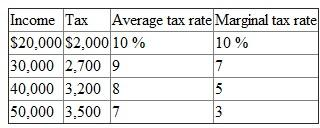# Quiz 18: Taxation and Resource Allocation

The marginal tax rate is calculated by considering the changes in the tax rates. Average tax rate is measured by considering the taxable income and the tax amount. The following is a table which indicates the measurement of marginal and average tax rate.In the above table, average tax rate changes according to changes in the tax amount and income level. An increase in income is greater than the increase in taxes. Therefore, average tax rate diminishes as income rises. Marginal tax rate also declines considering an increase in the level of income. The average tax rate diminishes as there is an increase in the income level. Therefore, the tax is considered as regressive. In terms of regressive tax, the average tax rate diminishes according to an increase in the level of income.